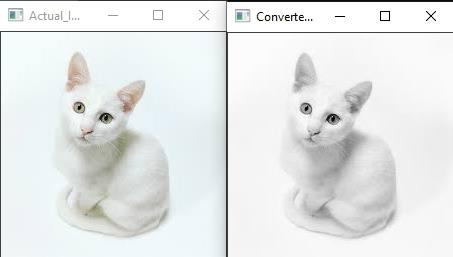# How to convert color spaces in OpenCV using C++?

OpenCVC++Server Side ProgrammingProgramming

#### OpenCV Complete Dummies Guide to Computer Vision with Python

70 Lectures 9 hours

#### Computer vision: OpenCV Fundamentals using Python

41 Lectures 4 hours

#### Python - OpenCV & PyQT5 together

51 Lectures 8 hours

Color space is the model of representing colors. There are different ways of describing colors. For example, RGB, CYMK, HSV, Grayscale etc.

Here, we used a new header named 'imgproc.hpp'. This 'imgproc.hpp' is the abbreviation of Image Processing. To convert color spaces, we need to use 'cvtColor()' function of OpenCV. This function is defined in 'imgproc' header file. That's why we have included 'imgproc.hpp'.

Firstly, we declared two matrices and two windows. These are for loading and showing the images. Then we loaded our image named 'cat.jpg' into 'myImage' matrix.  After that we used 'cvtColor(myImage, myImage_Converted, COLOR_RGB2GRAY)'. This line converts the RGB color space of 'myImage' to Grayscale and stores it in 'myImage_Converted' matrix.

The raw form of the 'cvtColor() function is −

cvtColor(Source Matrix, Destination Matrix, Color Space Conversion Code)

In this program, the source matrix is 'myImage', destination matrix is 'myImage_Converted', and color space conversion code is COLOR_RGB2GRAY.

The following program converts RGB images to Grayscale images in OpenCV.

## Example

#include<iostream>
#include<opencv2/highgui/highgui.hpp>
#include<opencv2/imgproc/imgproc.hpp>
using namespace cv;
using namespace std;
int main(int argc, const char** argv) {
Mat myImage;//declaring a matrix to load the image//
Mat myImage_Converted;//declaring a matrix to store the converted image//
namedWindow("Actual_Image");//declaring window to show actual image//
namedWindow("Converted_Image");//declaring window to show converted image//
}